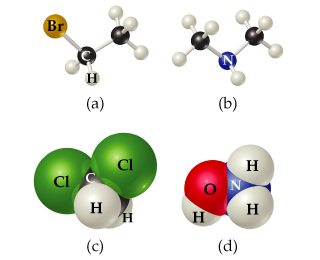# Problem: Write the molecular and structural formulas for the compounds represented by the models in the figure.Write the structural formula for the compound (d), assuming that all bonds are single bonds.

###### FREE Expert Solution

structural formula → shows arrangement of atoms in the compound

88% (178 ratings)###### Problem Details

Write the molecular and structural formulas for the compounds represented by the models in the figure.Write the structural formula for the compound (d), assuming that all bonds are single bonds.

Frequently Asked Questions

What scientific concept do you need to know in order to solve this problem?

Our tutors have indicated that to solve this problem you will need to apply the Molecular Models concept. If you need more Molecular Models practice, you can also practice Molecular Models practice problems.